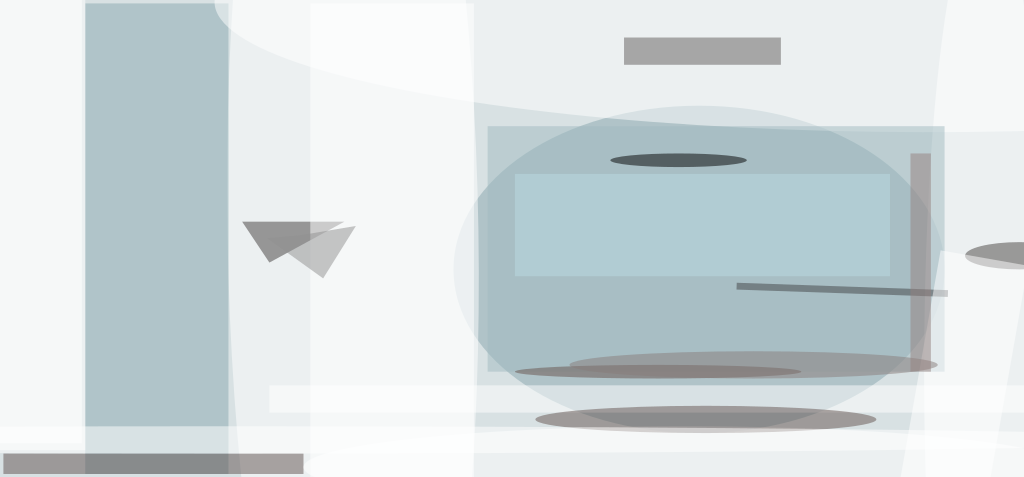An explicit material model that uses predicted pressure in the pores of a carbon-cloth phenolic (CCP) composite has been developed. This model is intended to be used within a finite-element model to predict phenomena specific to CCP components of solid-fuel-rocket nozzles subjected to high operating temperatures and to mechanical stresses that can be great enough to cause structural failures. Phenomena that can be predicted with the help of this model include failures of specimens in restrained-thermal-growth (RTG) tests, pocketing erosion, and ply lifting.

Heretofore, an implicit formulation has been used to model the pore pressure. The differences between explicit and implicit models can be illustrated with the theoretical solution for stress and strain in an RTG test. The equations for the explicit case are:

• σx = −αExΔT + (2vxw − 1)σp,
• εθ = (αw + vxwαxT + (1 − 2vxwvwxvwfp/Ew, and
• σw = σf = σp

where

• σx is the measured axial stress,
• εθ is the measured lateral (circumferential) strain,
• σw is the warp stress,
• σf is the fill stress,
• σp is the pore stress,
• αx is the across-ply coefficient of thermal expansion (CTE),
• αw is the warp CTE,
• ΔT is the change in temperature,
• Ex is the across-ply modulus of elasticity,
• Ew is the warp modulus of elasticity,
• vxw is the (across-ply)-warp Poisson's ratio,
• vwx is the warp-(across-ply) Poisson's ratio, and
• vwf is the warp-fill Poisson's ratio.An RTG Test is performed on a cylindrical specimen made of plies stacked along the axis and heated uniformly. The axial load and lateral strain are recorded versus temperature. A LHMEL test is performed on 1.5-by-1.5-by-0.75-in. (3.8-by-3.8-by-1.9-cm) specimen that is restrained on all sides except one, which is irradiated with a laser beam at a heating rate equivalent to that in an operating rocket nozzle. The temperature and pore pressure are measured at various distances from the irradiated surface.

For the implicit case, the σp term is zero. The most obvious implication of this is that the fiber stresses (σw, σf) would also be zero for an implicit material model. At the same time, fiber failure is known to occur during RTG testing. Thus, the failure of RTG specimens cannot be predicted with an implicit material model, but can with an explicit model.

One aspect of the development of the explicit model was to ensure that the properties used in the equations came from tests performed at low heating rates, so that effects of pore pressure could be considered separately from other effects. Another aspect of the development of the explicit model is the use of an additional equation for the pore stress:

• σp = Ppη,

where Pp is the pore pressure and η is the porosity of the CCP material. Here, the pore stress is regarded as the stress induced in the structure by the pore pressure. Because Pp had not been measured in an RTG configuration, for the purpose of testing and comparison, a pore-stress distribution versus temperature was assumed, then modified to correlate with measured RTG data. The only information known about the pore stress was that many RTG specimens had exhibited fiber failure at temperatures from 750 to 900 °F (399 to 482 °C). Knowing that the fiber stress equals the pore stress and the fiber tensile capability in this temperature range, it is possible to calculate a pore-stress data point.

The explicit and implicit models were compared in analyses of data from (1) RTG tests (2) high-heating-rate tests of a different type called "LHMEL" because they were performed in a facility called the "Laser Hardened Materials Evaluation Laboratory." The figure depicts the basic RTG and LHMEL configurations. Both models were found to be equally capable of predicting the axial stresses and lateral strains measured in the RTG tests. The explicit model was found to surpass the implicit one by being able to predict a reasonable fiber stress. On the basis of fiber stress, the explicit model can predict failures of RTG specimens.

Both models were also found to predict similar results for most quantities analyzed with respect to the LHMEL tests. The explicit model was found to surpass the implicit one by being able to predict reasonable mechanical strain and stress in the warp direction. This ability leads to the prediction of pocketing erosion in LHMEL tests. Moreover, the explicit model can also be used to indicate the depth, temperature, and time of occurrence of a pocket.

The analyses revealed that the predictions of the implicit and explicit models are similar except in the cases of certain stress and strain components associated with free expansion under a thermal load. For prediction by the explicit model, these stress components have been shown to be useful for predicting material failures of a CCP used in a solid-fuel rocket motor. Such failures cannot be predicted as easily, if at all, by use of the implicit model. The only major disadvantage of the explicit model is that in order to use it, one must have accurate values of pore pressure, data from low-heating-rate tests, and porosity; standard procedures for measuring these quantities have not yet been established.

This work was done by Danton Gutierrez-Lemini and Curt Ehle of Thiokol Corp., Inc., for Marshall Space Flight Center.

For further information, contact Sammy Nabors, MSFC Commercialization Assistance Lead, at This email address is being protected from spambots. You need JavaScript enabled to view it.. Refer to MFS-31501.##### NASA Tech Briefs Magazine

This article first appeared in the July, 2003 issue of NASA Tech Briefs Magazine.

Read more articles from the archives here.